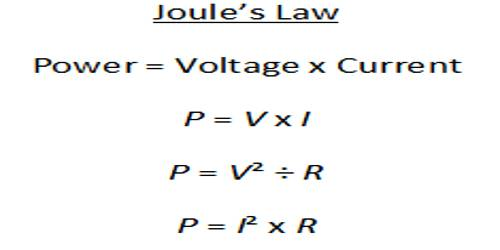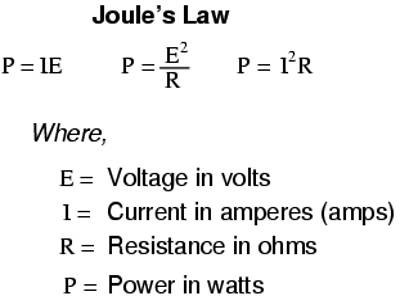# Joule’s Laws of Heat

Joule’s Laws of Heat

Joule heating describes the process where the energy of an electric current is converted into heat as it flows through a resistance.

Joule’s laws: There are three laws of Joule for the generation of heat:

(1) If the resistance of the conductor and time of flow of current remains constant, then the heat generated due to the flow of current is directly proportional to the square of the current.

(2) If the current and time of flow remain constant than heat generated in a conductor due to the flow of current is directly proportional to the resistance of the conductor.

(3) If the resistance of a conductor and flow of current through it remain constant, then the heat generated in the conductor due to the tone of flow of current is directly proportional to the time of flow of current.A quantitative form of Joule’s law is that the heat evolved per second, or the electric power loss, P, equals the current I squared times the resistance R or P = I2R. The power P has units of watts, or joules per second when the current is expressed in amperes and the resistance in ohms.